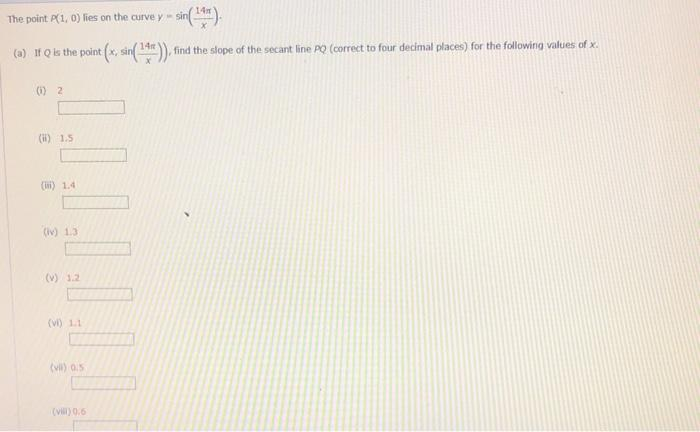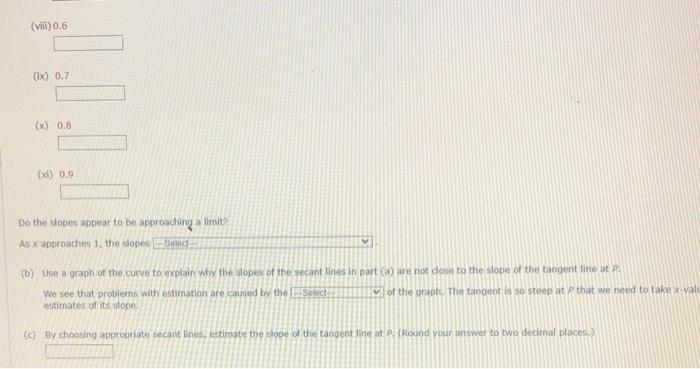Home / Expert Answers / Calculus / the-point-p-1-0-lies-on-the-curve-y-sin-left-frac-14-pi-x-right-a-if-q-pa580

# (Solved): The point $$p(1,0)$$ lies on the curve $$y=\sin \left(\frac{14 \pi}{x}\right)$$. (a) If $$Q$$ ...The point $$p(1,0)$$ lies on the curve $$y=\sin \left(\frac{14 \pi}{x}\right)$$. (a) If $$Q$$ is the point $$\left(x, \sin \left(\frac{14 \pi}{x}\right)\right)$$, find the slope of the secant line $$P Q$$ (correct to four decimal places) for the following values of $$x$$. (i) 2 (ii) $$1.5$$ (iii) $$1.4$$ (iv) $$1.3$$ (v) $$1.2$$ (vi) $$1.1$$ (*il) $$0.5$$ (vim) 0.6 Do the ilopes appear to be approaching a fimit? As $$x^{\text {aporeaches } 1, \text { the fopes }}$$ (b) Use a graph of the curve to explain why the sopes of the secant lines in part (a) ark not dose to the stope of the tangent fine at P. We see that problems with estimation are carsed by the estimates of its slope: (c) By choosing appropriate secant lines, estimate the sfope of the tangent line at P. (Round your aniswer to two decimal placess?

We have an Answer from Expert